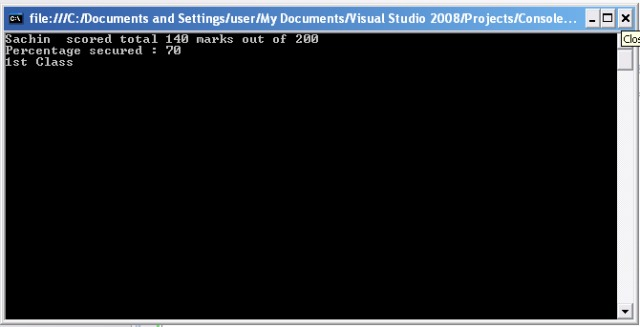After having a look at introduction to C#, let’s wear the programmer’s gloves and code something. For coding any program, the integral part of the code is its variables and data type of that particular variable,

C# supports following basic data types:

• Integer
• Floating point
• String
• Boolean

Integer variable can be defined using various subset data types available as per requirement size.

 Keywords Range Memory allocated Sbyte -128 to 127 1 byte byte 0 to 255 1 byte char 1 character(Unicode format) 2 byte short -32,768 to 32,767 2 byte ushort 0 to 65,535 2 byte int -2,147,483,648 to 2,147,483,647 4 byte uint 0 to 4,294,967,295 4byte long -9,223,372,036,854,775,808 to 9,223,372,036,854,775,807 8 byte ulong 0 to 18,446,744,073,709,551,615 8 byte

Example: int age = 21;

Floating point variables can be defined using following keywords :

 Keywords Range Memory allocated Precision Float ±1.5 × 10−45 to ±3.4 × 1038 4 byte 7 digits Double ±5.0 × 10−324 to ±1.7 × 10308 8 byte 15 digits decimal 1 character(Unicode format) 16 byte 29 digits

Example: float percentage = 98.99;

String keyword is used to declare a variable which contains a set of characters enclosed in double-quotes(” “). It may contain a combination of integers with alphabet.

Example : string s = “ABC”;

Boolean data type is used to store the either of the two values i.e True or False. It is majorly used in loops and decision making statements.

Example: bool flag = true;

The following example displays the name, total marks  and  percentage secured.

using System;
using System.Collections.Generic;
using System.Linq;
using System.Text;namespace ConsoleApplication1
{
class Program
{
static void Main(string[] args)
{
String name = “Sachin“;                         //String variable
int sub1 = 65;                                            //int variable
int sub2 = 75;
int total = sub1 + sub2;
float per = (total / 200) * 100;                     //float variable
Console.WriteLine(name+”  scored total “+ total + “marks out of 200 “);
Console.WriteLine(“Percentage secured : ” + per);
if (per > 60)
{
Console.WriteLine(“1st Class“);
}
else
Console.WriteLine(“2nd Class“);